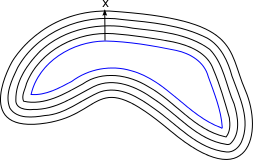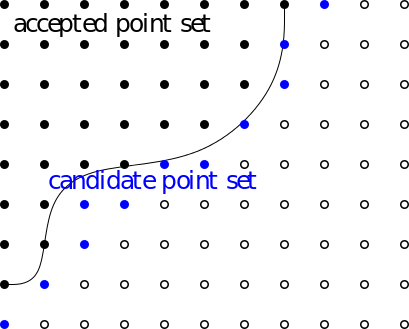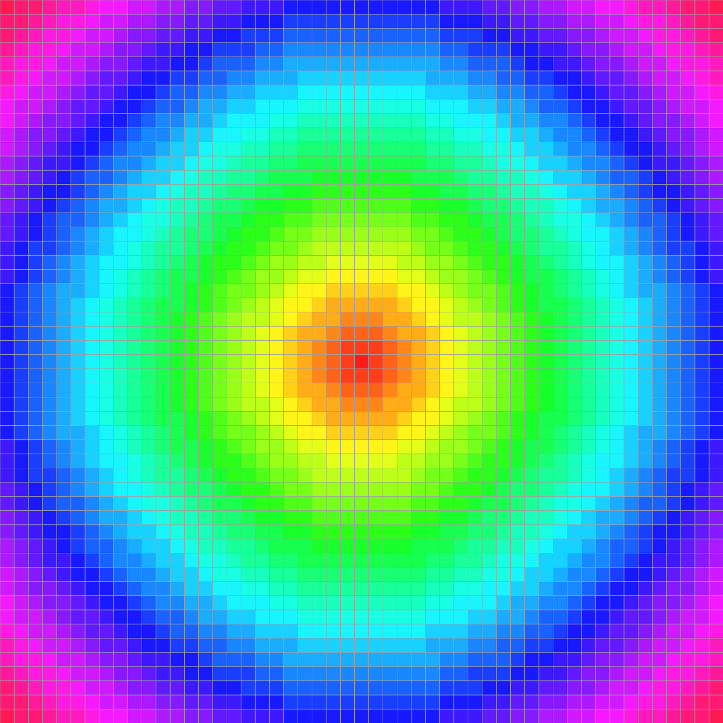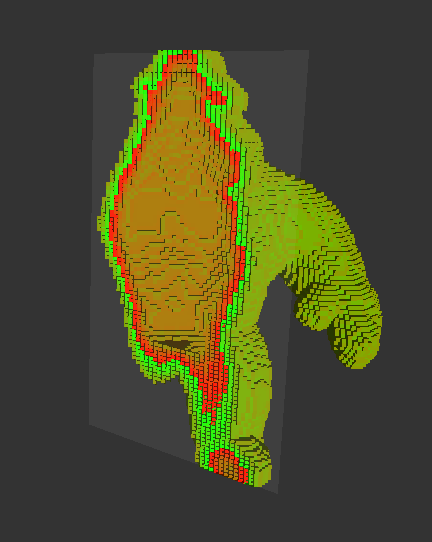DGtal  1.4.beta
nD Fast Marching Methods

Author(s) of this documentation:
Tristan Roussillon

Part of the Geometry package.

The Fast Marching Method (FMM for short) is a numerical technique for tracking the evolution of an expanding front within the level-set framework (see  for a review).
If the front evolves in the normal direction at unit speed, the arrival time at a point $$x$$ corresponds to the distance of $$x$$ to the initial front.Arrival time and distance to the initial front

That is why FMM can be used to compute a (signed) distance field from an initial point set (which may be located around an interface or not).
However, FMM can be used to other tasks: e.g. the extrapolation of a scalar field out of an interface, such that it is constant on rays normal to the interface .

Related examples are exampleFMM2D.cpp and exampleFMM3D.cpp.

# Overview

Let us assume that we compute in the fundamental lattice $$\mathbb{Z}^n$$ the distance field from a given lattice point set. The distance value of each lattice point is computed by marching out from the initial set of lattice points for which the distance values are known. This set is an initialization of the so-called accepted point set, which incrementally grows until all lattice points of the computation domain have been accepted or until a stopping criterion has been met.
Each lattice point adjacent to one of the accepted points is put into the so-called candidate point set.

The accepted point set and the candidate point set are depicted below. See Data structures for details about how these sets are stored.Upwind update of the accepted point set

For each candidate point, a tentative value is computed for its distance, using only the values of the accepted points lying in its neighborhood. This task is delegated to an instance of a model of CPointFunctor, which is defined as L2FirstOrderLocalDistance by default. However, you are free to use L2SecondOrderLocalDistance, for second order convergence, L1LocalDistance and LInfLocalDistance for other norms. See Computing distances for further details.

Then, the point of smallest tentative value is iteratively added to the set of accepted points. The tentative values of the candidates adjacent to the newly added point are updated using the distance value of the newly added point.

## Data structures

The class FMM, which implements the fast marching method, is based on two external data structures and one internal data structure:

• External data structures:
• an instance of a model of CDigitalSet for the accepted point set.
• an instance of a model of CImage, which stores the distance values.

In practice, you can choose either ImageContainerBySTLVector and DigitalSetBySTLSet if you work on the whole domain:

typedef ImageContainerBySTLVector<Domain,double> DistanceImage;
typedef DigitalSetBySTLSet<Domain> AcceptedPointSet;

or ImageContainerBySTLMap and DigitalSetFromMap if you work on a small fraction of the domain:

typedef ImageContainerBySTLMap<Domain,double> DistanceImage;
typedef DigitalSetFromMap<DistanceImage> AcceptedPointSet;
• Internal data structure:
• a data structure for the candidate point set. Three operations are required:

• insert a new point with its distance value,
• find a given point, update its tentative value if found,
• get the point of smallest tentative value,

This data structure is usually a min-heap data structure with back pointers from the lattice to the min-heap (see ). The memory cost of such solution is however high. That is why, we implemented the candidate point set as a STL set of pairs <point, tentative value>. Instead of updating the tentative values, we insert a new pair <point, tentative value>. This solution is less memory consumming and experimentally (nearly) as efficient as the former one.

## Computing distances

In a numerical point of view, the key point of the algorithm is to accurately approximate the distance value of a given point from the distance value of its neighbors.

Four models of CPointFunctor can be used for this task:

We detail below how L2FirstOrderLocalDistance, which is used by default, approximate the Euclidean distance at some point $$x$$, from the available distance values of the lattice points lying in the 1-neighborhood of $$x$$.

The distance value is computed such that the upwind gradient of the distance image is one. Let us denote by $$d$$ the distance value at $$x$$. Moreover, for a given quadrant, let us denote by $$d_i$$ the distance value of the unique 1-neighbor of $$x$$ along the $$i$$ axis. The distance value $$d$$ is taken as the minimum solution of the following quadratic equation

$$\sum_{i = 1 \ldots n } ( d - d_i )^2 = 1$$

Obviously, if the distance value $$d'$$ of only one 1-neighbor is available, then $$d = d' \pm 1$$ ( $$+$$ for positive distance values, $$-$$ for negative ones).
If the distance values of two (or more than two in nD) 1-neighbors are available, the previous quadratic equation should have two solutions and we take the larger one for $$d$$.
The numerical approach implemented in L2FirstOrderLocalDistance::operator() to compute $$d$$, even with poor initial data or with numerical errors, is detailed in  [p. 72-73]. Note that if $$h$$ is the lattice spacing, the approximation error tends to zero in $$O(h)$$ (first-order convergence).

Note
This class deals with positive or negative distance values (0 is arbitrarily considered as a positive value, ie. starting with a seed of null value, you must get positive values). However, the behavior is undefined when there are both positive and negative distance values in the neighborhood of $$x$$. See Signed distance field to an interface
for an example of the use of signed distance values.

## Basic usage

In DGtal, the class FMM is parametrized by the following types:

After the inclusion of the following file

#include "DGtal/geometry/volumes/distance/FMM.h"

you may define the FMM type as follows:

typedef ImageContainerBySTLMap<Z2i::Domain,double> DistanceImage;
typedef DigitalSetFromMap<DistanceImage> AcceptedPointSet;
typedef Z2i::Domain::Predicate DomainPredicate;
typedef FMM<DistanceImage, AcceptedPointSet, DomainPredicate> FMM;
functors::IsWithinPointPredicate< Point > Predicate

Since an instance of FMM keeps a reference to the accepted point set, a reference to the distance image, and a constant reference to the point predicate, you must be sure that these three objects have been constructed and exist before constructing a FMM instance.

DistanceImage distanceImage( domain );
AcceptedPointSet set( distanceImage );
static Self diagonal(Component val=1)
HyperRectDomain< Space > Domain
Definition: StdDefs.h:99
Domain domain

In this example, we start from one point whose distance value is zero:

set.insert( origin );
distanceImage.setValue( origin, 0.0 );
Space::Point Point
Definition: StdDefs.h:95

We now construct a FMM instance as follows:

FMM fmm( distanceImage, set, domain.predicate() );
Warning
Since the candidate point set is initialized at construction (see FMM::init()), you must construct the initial set of accepted points before the construction of the FMM instance. If the accepted point set is empty at construction, a DGtal::InputException is raised.

After the call of the method FMM::compute(), the distance value of all the accepted points is available in the distance image.

fmm.compute();
Warning
You must be sure that the point predicate implicitly represents a finite point set that is included in the image domain. Otherwise, the computation may never end or may raise an execution error.

Here is the computed distance field:Approximation by the FMM of the Euclidean distance field from a given point
Note
The computed distance field is a (convergent) estimation. See nD Volumetric Analysis using Separable Processes for an exact Euclidean distance transform.

The whole example may be found in exampleFMM2D.cpp.

## Stopping criteria

The computation ends either if all the points represented by the point predicate have been accepted, or if one of the following stopping criteria have been met:

• the number of accepted points equals to a given threshold.
• the maximal distance (in absolute value) exceed a given threshold.

In order to use these stopping criteria, you must provide the corresponding thresholds at construction as follows:

FMM fmm( imageDistance, initialPointSet, domain.predicate(),
maximalNumberOfPoints, maximalDistance );

With the above code, we stop the computation as soon as the number of accepted points equals to maximalNumberOfPoints or the distance value of the next candidate point exceed maximalDistance.

Note that you can also perform the computation step by step. This approach may be useful to better control the computation and its end. You must use FMM::computeOneStep(), which takes as input and returns the last accepted point and its distance value, as follows:

Point lastPt = Point::diagonal(0); //the last accepted point...
double lastDist = 0.0; //and its distance value
while ( (fmm.computeOneStep( lastPt, lastDist ))
&& ( /* optional stopping criterion */ ) )
{
/* you can do something before the next step */
}
MyPointD Point
Definition: testClone2.cpp:383

# Applications

As reported in , the Fast Marching Method has numerous applications.

## Signed distance field to an interface <br>

A signed distance field can be used to

• compute geometrical quantities by differential operators: e.g. the mean curvature at a point $$x$$ of an interface
• implicitly evolve an interface: e.g. adding a scalar to a distance field is a way of moving each point of the interface in the normal direction with a constant speed (operation known as a dilation).

In exampleFMM3D.cpp, we show how to compute a signed distance field to a digital surface.

First, we define the FMM type as follows:

typedef ImageContainerBySTLMap<Domain,double> DistanceImage;
typedef DigitalSetFromMap<DistanceImage> AcceptedPointSet;
typedef Domain::Predicate DomainPredicate;
typedef FMM<DistanceImage, AcceptedPointSet, DomainPredicate > FMM;

Then, we construct and initialize the external data structures as follows:

DistanceImage imageDistance( domain, 0.0 );
AcceptedPointSet initialPointSet( imageDistance );
FMM::initFromBelsRange( ks, frontier.begin(), frontier.end(),
imageDistance, initialPointSet, 0.5 );
static void initFromBelsRange(const KSpace &aK, const TIteratorOnBels &itb, const TIteratorOnBels &ite, Image &aImg, AcceptedPointSet &aSet, const Value &aValue, bool aFlagIsPositive=true)

In the above snippet, we insert all the 3D lattice points incident to the digital surface in the initial point set and we assign to them an initial distance value (equal to $$0.5$$ for outer points and $$-0.5$$ for inner points) by merely calling the static method FMM::initFromBelsRange().

Note
There are several static methods that help to construct the initial point set and to provide a distance value for all the points of this set:

Finally, we instanciate the FMM class and we perform the whole computation:

FMM fmm( imageDistance, initialPointSet, domain.predicate(),
domain.size(), maximalDistance );
fmm.compute();
trace.info() << fmm << std::endl;
std::ostream & info()
Trace trace
Definition: Common.h:153

The computation is limited to a narrow band around the digital surface: each point of the band are located at a distance less than maximalDistance to the digital surface.

The result is displayed below.Signed distance field computed in a narrow band around a digital surface.